## #DS2TipWeek - Arranging Shapes Using Index

by Lorna Eden

This little trick is going to show you how to put custom shapes into two columns using index. Also this particular exercise will arrange them in Alphabetical order. If you need to refresh yourself on how to create custom shape filters click here.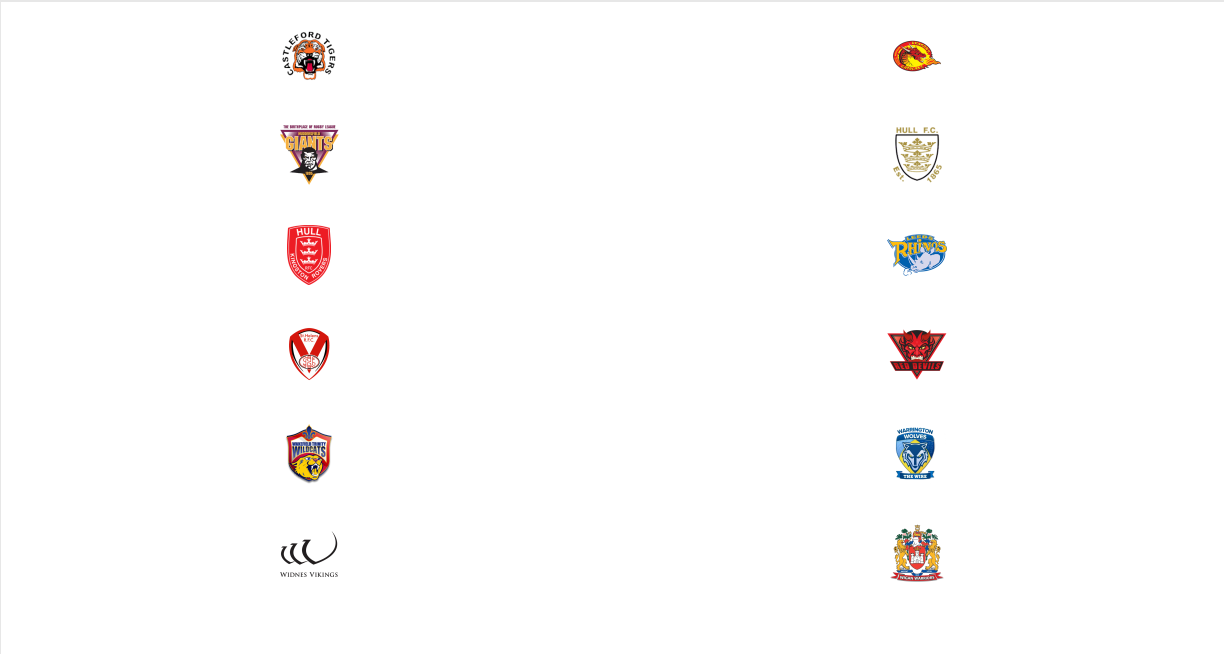So the first question you might ask is why would you need to do this, why not have one long list? Well the simple answer is space. You might not have that much space on a dashboard that requires you to make the shapes smaller etc. Using two columns allows this section to be smaller in height but can also be just as narrow as a single column.

Let’s start with a single column, as you can see the shapes are smaller because everything has to fit in this one view. If you put this in a dashboard the shapes would overlap and you would have to reduce the size.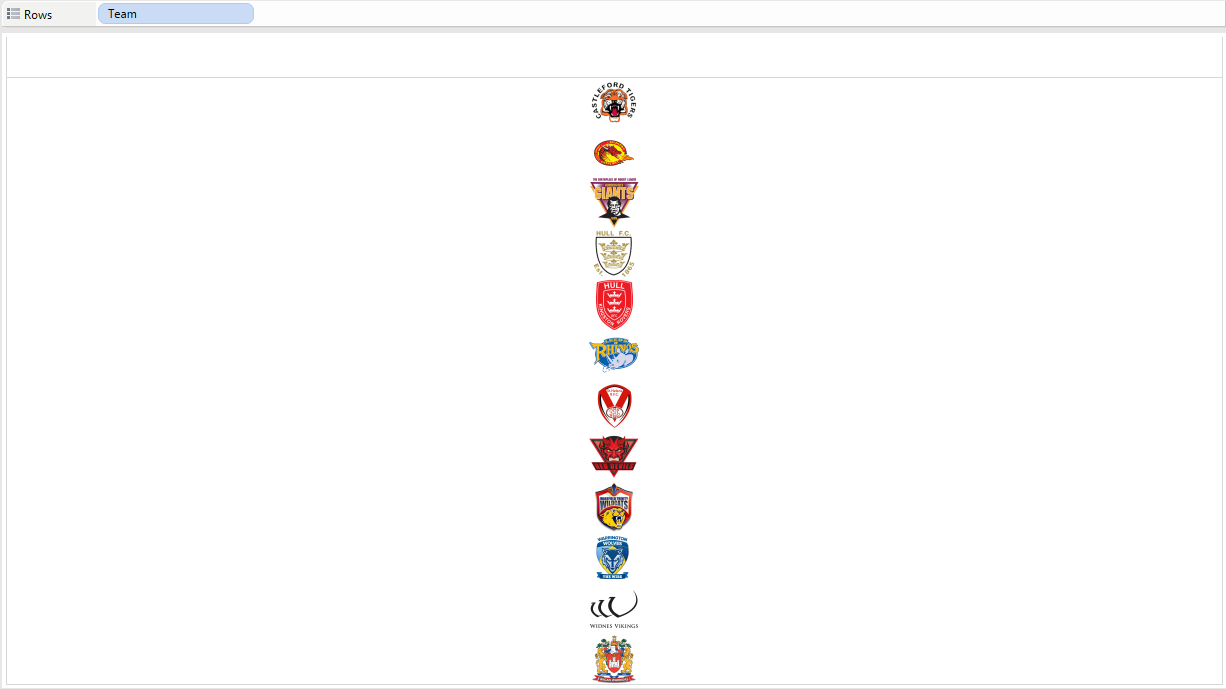We need to give each team a number related to the position show above. This function is called index. This first index function is saying if the index number is divisible by 2 with no leftovers (hence the =0) then move it back one row else just use the index.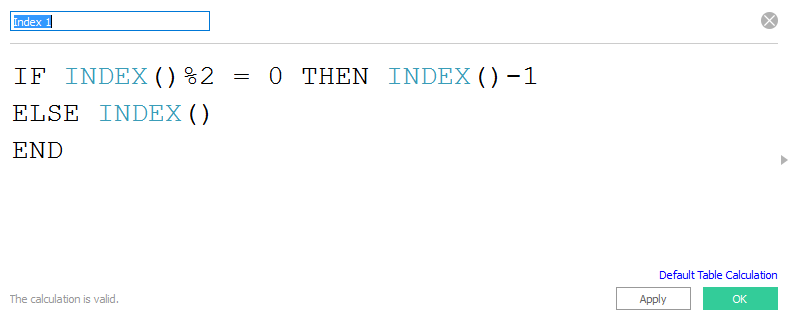If we move this calculation to rows and keep team to shape. It will look like this. Oh no it hasn’t worked… Wait we haven’t changed the compute using.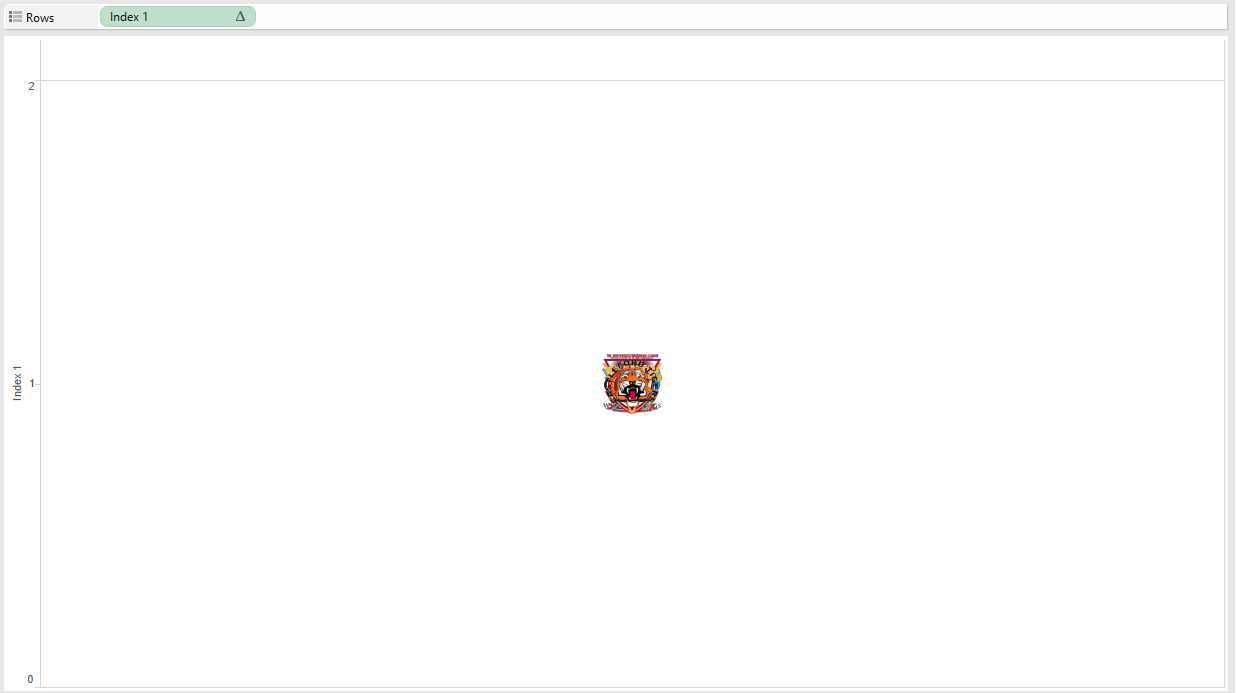We need to tell the calculation to compute using ‘team’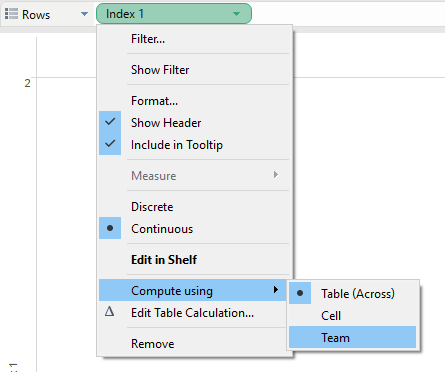This will then spread the teams out. As you can see there is two teams on top of each other. This is where the next equation comes in.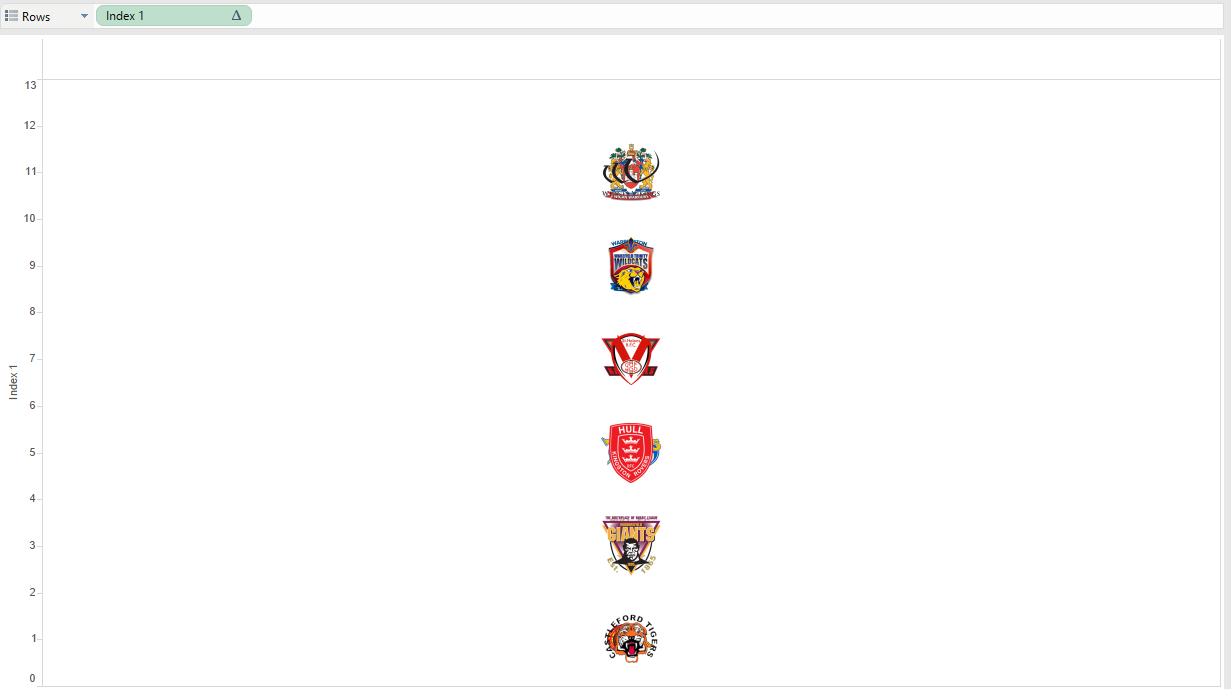The second index equation is basically assigning each team either 1 or 2 based on their alphabetical position. So we want all the odd number on the left. When you put this equation onto the columns shelf, change it to discrete and then also compute using team.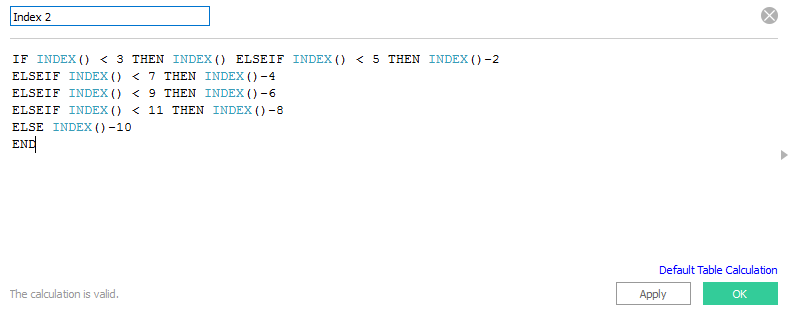Once you have done the steps above your icons should look like the one below.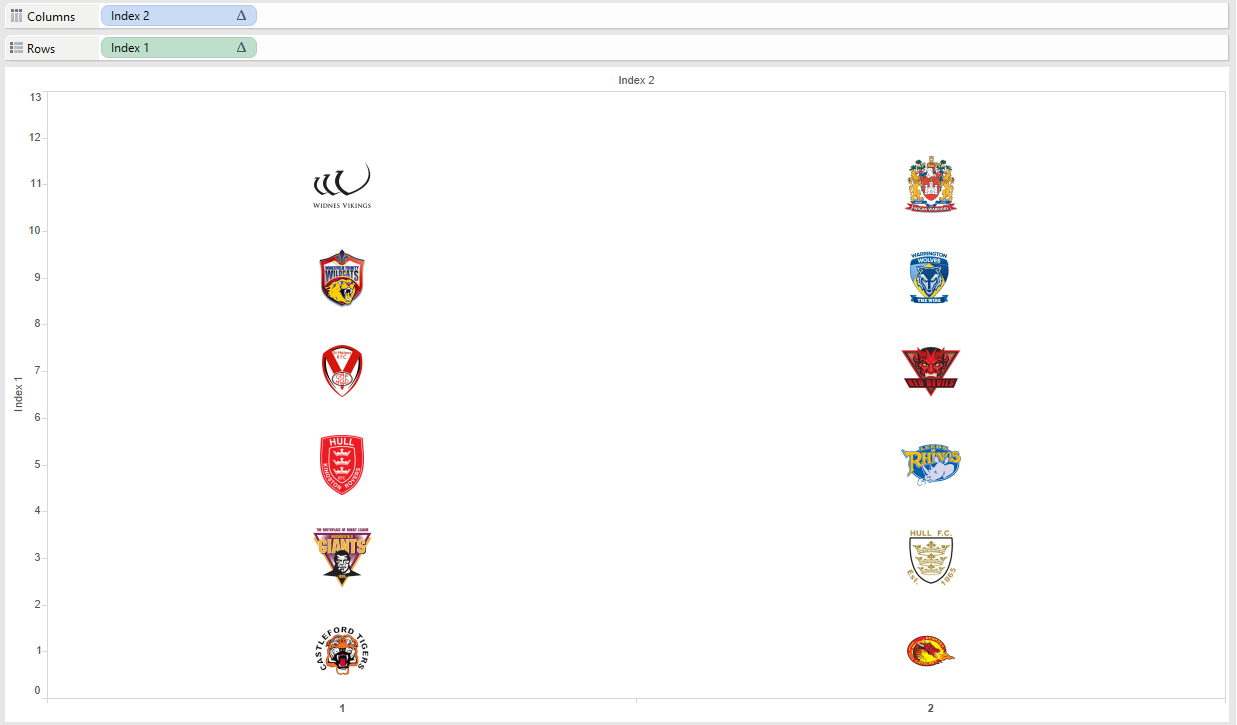Now we just need to reverse the y axis (Index 1), so that the first team is then back at the top.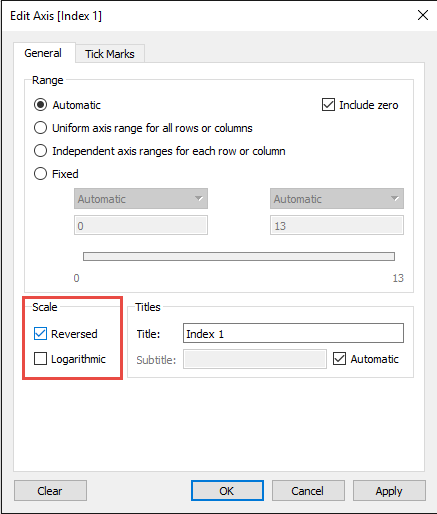Then finally just hide the headers on both rows and columns, then viola this is the end result.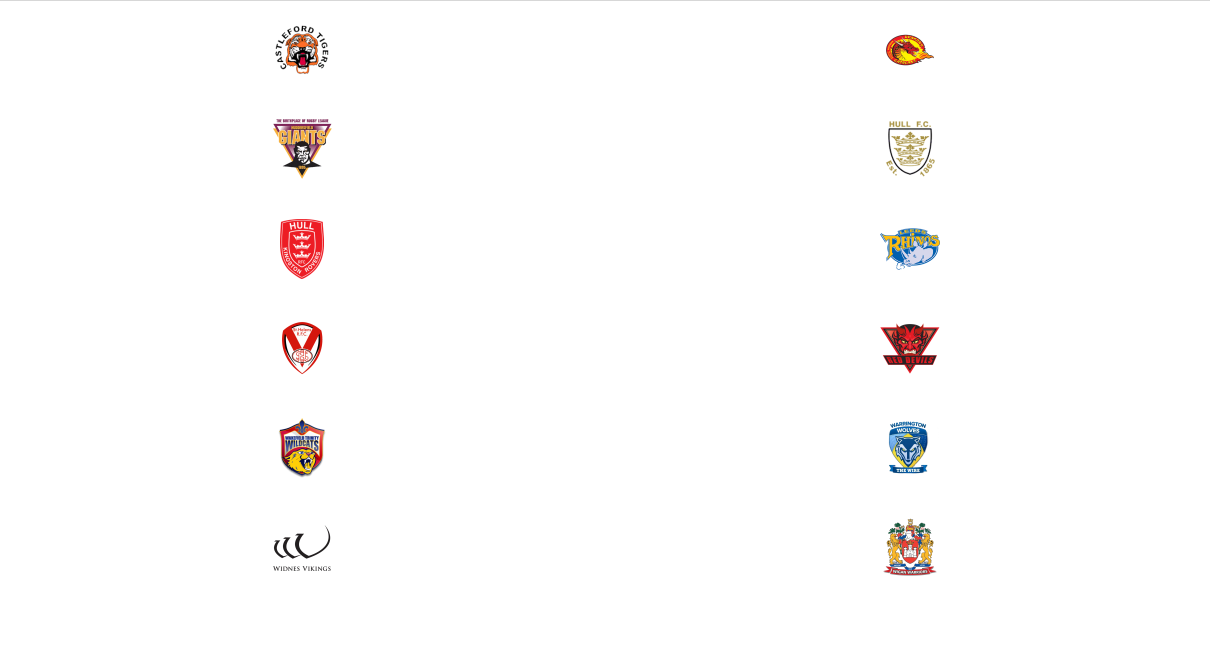Again credit to Coach Andy for helping me fix this issue.

Thanks for reading, let me know if you struggle with recreating this and I can help you sort the issue.Lorna Eden